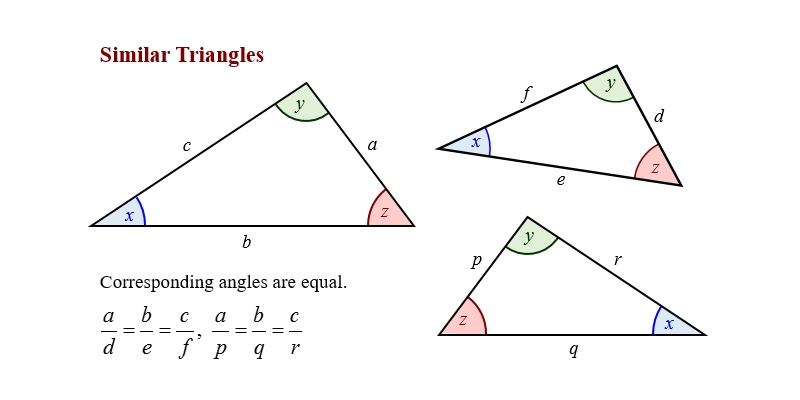### Similarity Of Triangle Trivia Quiz### Questions & Options

#### 1. Two similar triangles have ....

 112574 Equal sides 112575 Equal areas 112576 Equal angles 112577 All of these

#### 2. Two congruent triangles have ......

 112578 Propotional sides 112579 Equal sides 112580 Equal corresponding angles 112581 Equal corresponding sides

#### 3. If two triangles are on the same base and between the parallel lines then they will be.....

 112582 Equilaterals 112583 Equal in area 112584 Right angled 112585 Congruent

#### 4. If two corresponding sides and the angle between them of a triangle are equal to another triangle. Then the angles are .........

 112586 Congruent but not similar 112587 Neither congruent nor similar 112588 Congruent and similar 112589 None of these

#### 5. Ration areas of two similar triangles are equal to ......

 112590 Ratio of squares of the corresponding altitudes 112591 Ratio of squares of corresponding medians 112592 Both of these 112593 None of these

#### 6. If the areas of two similar triangles are equal then the triangles.......

 112594 Are congruent 112595 Have equal length of corresponding sides 112596 Both of these 112597 None of these

#### 7. If the sides of a triangle are 8cm, 12cm, and 15cmthen the angle is ....

 112598 Right angle 112599 Obtuse angle 112600 Acute angle 112601 None of these

#### 8. If corresponding angles of two triangles are equal, then they are known as .......

 112602 Obtuse angle 112603 Acute Angle 112604 Equiangular triangles 112605 Isosceles triangles

#### 9. Two sides in a triangle have the same length as two sides in the other triangle, and the included angles have the same measure are called.....

 112606 SAS Postulate 112607 AAS Postulate 112608 SSS Postulate 112609 ASA Postulate

#### 10. Each side of a triangle has the same length as a corresponding side of the other triangle is called.....

 112610 AAS Postulate 112611 SSS Postulate 112612 SAS Postulate 112613 None of these